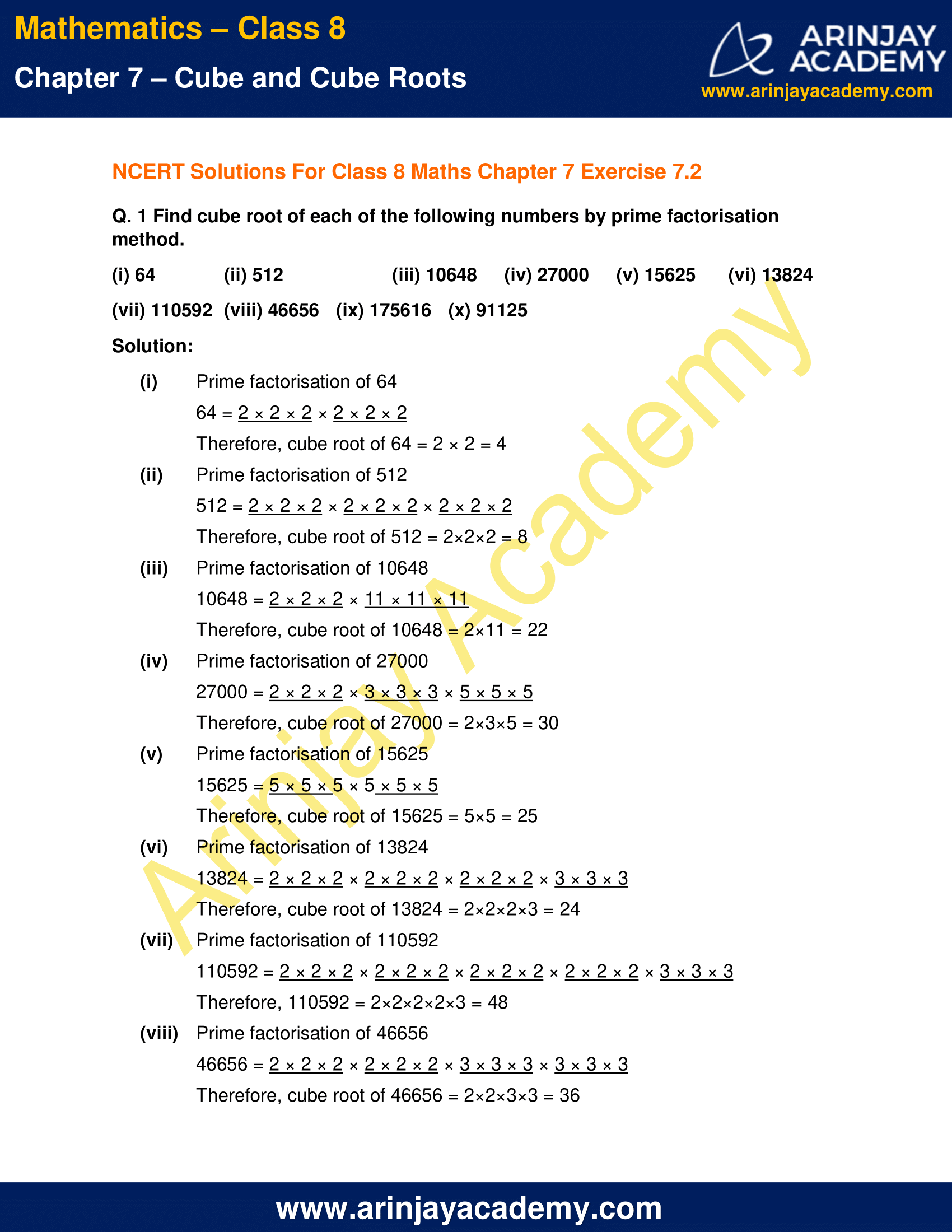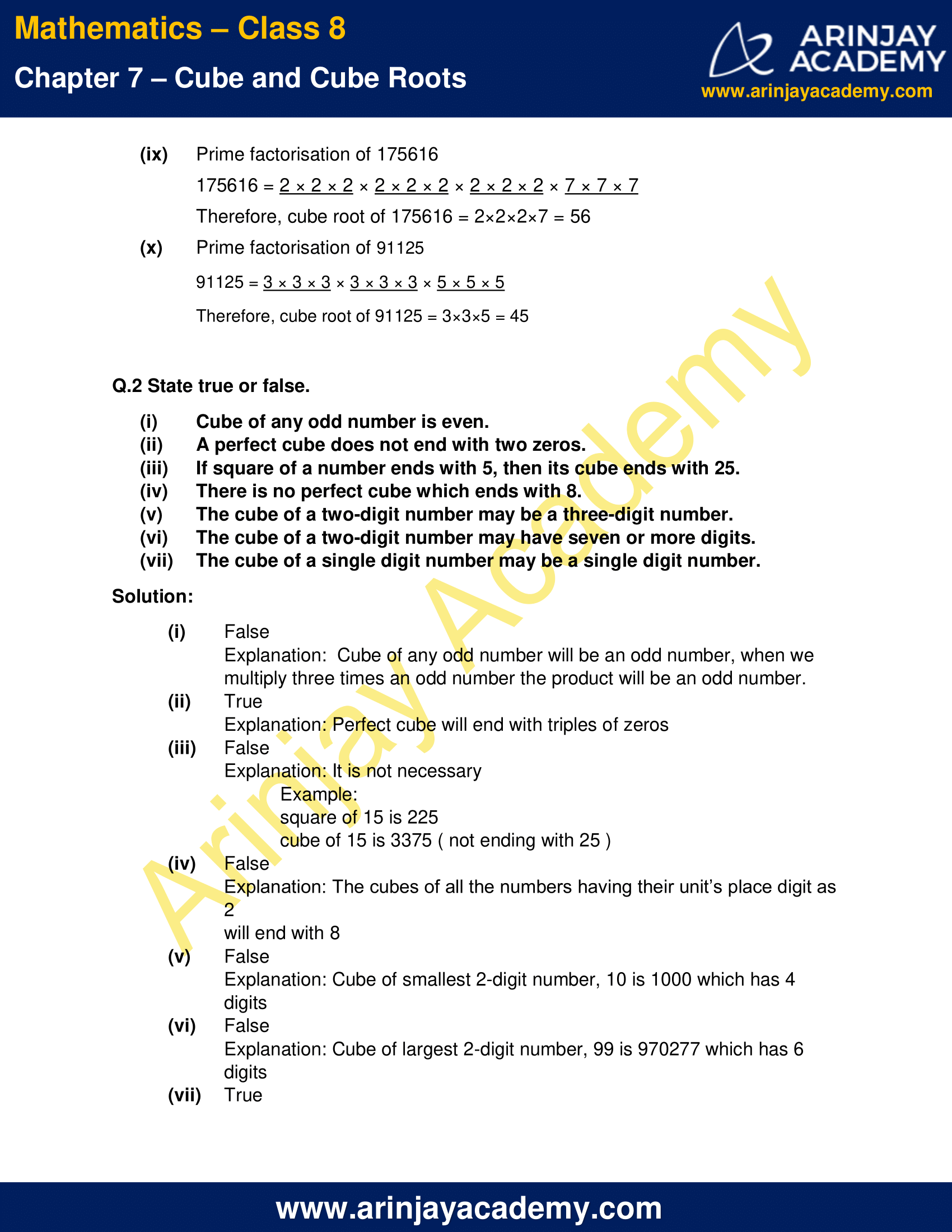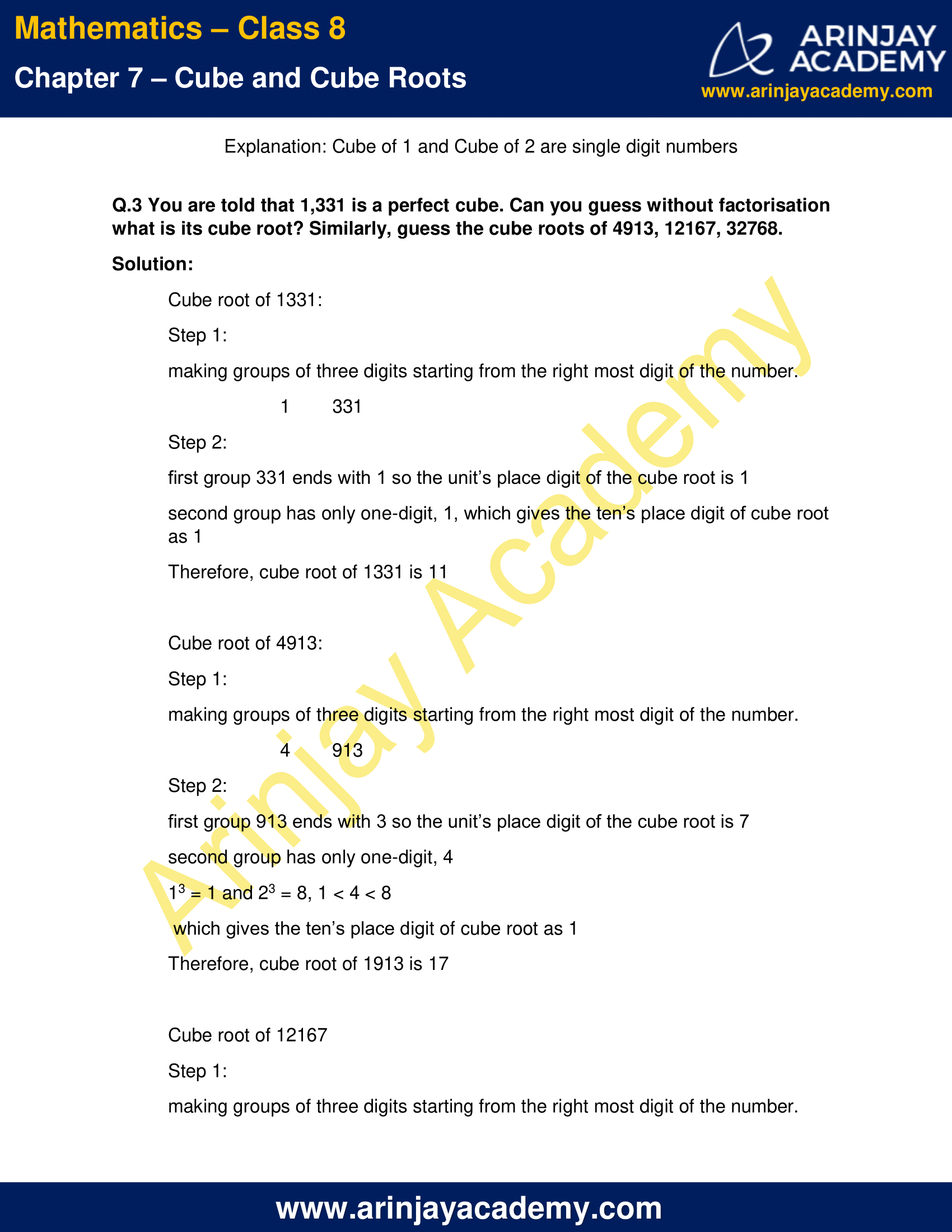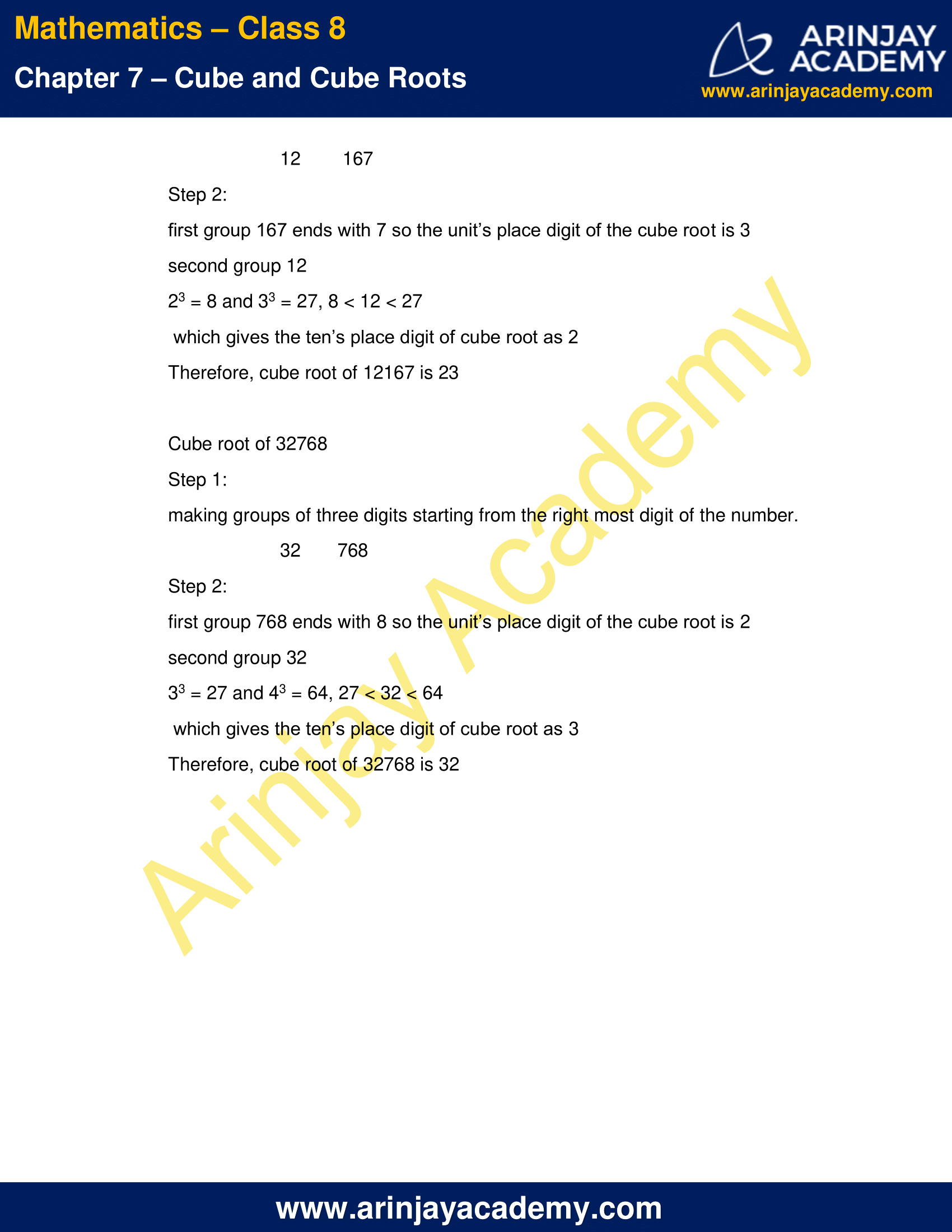# NCERT Solutions for Class 8 Maths Chapter 7 Exercise 7.2 – Cube and Cube roots

NCERT Solutions for Class 8 Maths Chapter 7 Exercise 7.2 – Cube and Cube roots, has been designed by the NCERT to test the knowledge of the student on the topic – Cube Roots

• Cube root through prime factorisation method
• Cube root of a cube number

### NCERT Solutions for Class 8 Maths Chapter 7 Exercise 7.2 – Cube and Cube rootsNCERT Solutions for Class 8 Maths Chapter 7 Exercise 7.2 – Cube and Cube roots

1.  Find cube root of each of the following numbers by prime factorisation method.

(i) 64
(ii) 512
(iii) 10648
(iv) 27000
(v) 15625
(vi) 13824
(vii) 110592
(viii) 46656
(ix) 175616
(x) 91125

Solution:

(i) Prime factorisation of 64
64 = 2 × 2 × 2 × 2 × 2 × 2
Therefore, cube root of 64 = 2 × 2 = 4

(ii) Prime factorisation of 512
512 = 2 × 2 × 2 × 2 × 2 × 2 × 2 × 2 × 2
Therefore, cube root of 512 = 2×2×2 = 8

(iii) Prime factorisation of 10648
10648 = 2 × 2 × 2 × 11 × 11 × 11
Therefore, cube root of 10648 = 2×11 = 22

(iv) Prime factorisation of 27000
27000 = 2 × 2 × 2 × 3 × 3 × 3 × 5 × 5 × 5
Therefore, cube root of 27000 = 2×3×5 = 30

(v) Prime factorisation of 15625
15625 = 5 × 5 × 5 × 5 × 5 × 5
Therefore, cube root of 15625 = 5×5 = 25

(vi) Prime factorisation of 13824
13824 = 2 × 2 × 2 × 2 × 2 × 2 × 2 × 2 × 2 × 3 × 3 × 3
Therefore, cube root of 13824 = 2×2×2×3 = 24

(vii) Prime factorisation of 110592
110592 = 2 × 2 × 2 × 2 × 2 × 2 × 2 × 2 × 2 × 2 × 2 × 2 × 3 × 3 × 3
Therefore, 110592 = 2×2×2×2×3 = 48

(viii) Prime factorisation of 46656
46656 = 2 × 2 × 2 × 2 × 2 × 2 × 3 × 3 × 3 × 3 × 3 × 3
Therefore, cube root of 46656 = 2×2×3×3 = 36

(ix) Prime factorisation of 175616
175616 = 2 × 2 × 2 × 2 × 2 × 2 × 2 × 2 × 2 × 7 × 7 × 7
Therefore, cube root of 175616 = 2×2×2×7 = 56

(x) Prime factorisation of 91125
91125 = 3 × 3 × 3 × 3 × 3 × 3 × 5 × 5 × 5
Therefore, cube root of 91125 = 3×3×5 = 45

Q.2 State true or false.

(i) Cube of any odd number is even.
(ii) A perfect cube does not end with two zeros.
(iii) If square of a number ends with 5, then its cube ends with 25.
(iv) There is no perfect cube which ends with 8.
(v) The cube of a two-digit number may be a three-digit number.
(vi) The cube of a two-digit number may have seven or more digits.
(vii) The cube of a single digit number may be a single digit number.

Solution:

(i) False
Explanation:  Cube of any odd number will be an odd number, when we multiply three times an odd number the product will be an odd number.

(ii) True
Explanation: Perfect cube will end with triples of zeros

(iii) False
Explanation: It is not necessary
Example:
square of 15 is 225
cube of 15 is 3375 ( not ending with 25 )

(iv) False
Explanation: The cubes of all the numbers having their unit’s place digit as 2 will end with 8

(v) False
Explanation: Cube of smallest 2-digit number, 10 is 1000 which has 4 digits

(vi) False
Explanation: Cube of largest 2-digit number, 99 is 970277 which has 6 digits

(vii) True
Explanation: Cube of 1 and Cube of 2 are single digit numbers

Q.3 You are told that 1,331 is a perfect cube. Can you guess without factorisation what is its cube root? Similarly, guess the cube roots of 4913, 12167, 32768.

Solution:

Cube root of 1331:
Step 1:
making groups of three digits starting from the right most digit of the number.
1        331
Step 2:
first group 331 ends with 1 so the unit’s place digit of the cube root is 1
second group has only one-digit, 1, which gives the ten’s place digit of cube root as 1
Therefore, cube root of 1331 is 11

Cube root of 4913:

Step 1:
making groups of three digits starting from the right most digit of the number.
4        913
Step 2:
first group 913 ends with 3 so the unit’s place digit of the cube root is 7
second group has only one-digit, 4
13 = 1 and 23 = 8, 1 < 4 < 8
which gives the ten’s place digit of cube root as 1
Therefore, cube root of 1913 is 17

Cube root of 12167

Step 1:
making groups of three digits starting from the right most digit of the number.
12        167
Step 2:
first group 167 ends with 7 so the unit’s place digit of the cube root is 3
second group 12
23 = 8 and 33 = 27, 8 < 12 < 27
which gives the ten’s place digit of cube root as 2
Therefore, cube root of 12167 is 23

Cube root of 32768

Step 1:
making groups of three digits starting from the right most digit of the number.
32       768
Step 2:
first group 768 ends with 8 so the unit’s place digit of the cube root is 2
second group 32
33 = 27 and 43 = 64, 27 < 32 < 64
which gives the ten’s place digit of cube root as 3
Therefore, cube root of 32768 is 32

The next Exercise for NCERT Solutions for Class 8 Maths Chapter 8 Exercise 8.1 – Comparing Quantities can be accessed by clicking here